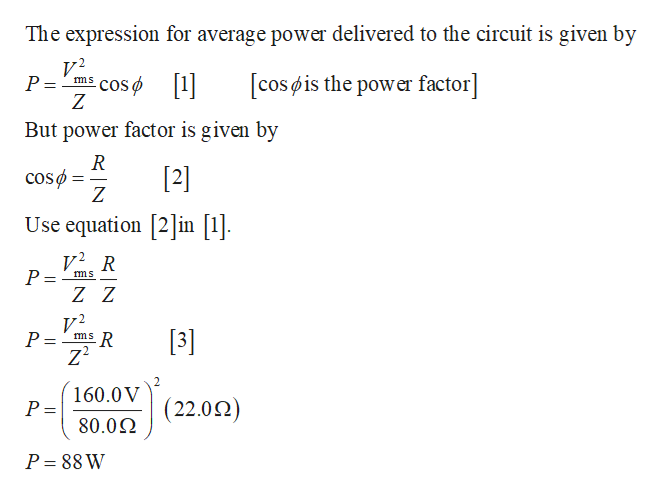# A series RLC circuit has a resistance of 22.0 Ω and an impedanceof 80.0 Ω. If the rms voltage applied to the circuit is160. V, what average power is delivered to the circuit?

Question
74 views

A series RLC circuit has a resistance of 22.0 Ω and an impedance
of 80.0 Ω. If the rms voltage applied to the circuit is
160. V, what average power is delivered to the circuit?

check_circle

Step 1

Write the expression fo...help_outlineImage TranscriptioncloseThe expression for average power delivered to the circuit is given by V2 P = ms cos ø [cos øis the power factor] 1| But power factor is given by R cosø =  Use equation in . v²¸ R P = V? P = -2  160.0V P = (22.02) 80.02 P = 88 W fullscreen

### Want to see the full answer?

See Solution

#### Want to see this answer and more?

Solutions are written by subject experts who are available 24/7. Questions are typically answered within 1 hour.*

See Solution
*Response times may vary by subject and question.
Tagged in

### Physics